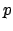# Galois Representations and a Conjecture of Artin

The Galois groupis an object of central importance in number theory, and I've often heard that in some sense number theory is the study of this group. A good way to study a group is to study how it acts on various objects, that is, to study its representations.

Endowwith the topology which has as a basis of open neighborhoods of the origin the subgroups, wherevaries over finite Galois extensions of. (Note: This is not the topology got by taking as a basis of open neighborhoods the collection of finite-index normal subgroups of.) Fix a positive integerand letbe the group ofinvertible matrices overwith the discrete topology.

Definition 14.3.1   A ofis a continuous homomorphismForto be continuous means that there is a finite Galois extensionsuch thatfactors through:For example, one could taketo be the fixed field of. (Note that continous implies that the image ofis finite, but using Zorn's lemma one can show that there are homomorphismswith finite image that are not continuous, since they do not factor through the Galois group of any finite Galois extension.)

Fix a Galois representationand a finite Galois extensionsuch thatfactors through. For each primethat is not ramified in, there is an elementthat is well-defined up to conjugation by elements of. This means thatis well-defined up to conjugation. Thus the characteristic polynomialis a well-defined invariant ofand. Letbe the polynomial obtain by reversing the order of the coefficients of. Following E. Artin, set(14.2)

We view.as a function of a single complex variable. One can prove thatis holomorphic on some right half plane, and extends to a meromorphic function on all.

Conjecture 14.3.2 (Artin)   The-series of any continuous representationis an entire function on all, except possibly at.

This conjecture asserts that there is some way to analytically continueto the whole complex plane, except possibly at. (A standard fact from complex analysis is that this analytic continuation must be unique.) The simple pole atcorresponds to the trivial representation (the Riemann zeta function), and ifandis irreducible, then the conjecture is thatextends to a holomorphic function on all.

The conjecture follows from class field theory forwhen. Whenand the image ofinis a solvable group, the conjecture is known, and is a deep theorem of Langlands and others (see [Lan80]), which played a crucial roll in Wiles's proof of Fermat's Last Theorem. Whenand the projective image is not solvable, the only possibility is that the projective image is isomorphic to the alternating group. Becauseis the symmetric group of the icosahedron, these representations are called . In this case, Joe Buhler's Harvard Ph.D. thesis gave the first example, there is a whole book [Fre94], which proves Artin's conjecture for 7 icosahedral representation (none of which are twists of each other). Kevin Buzzard and I (Stein) proved the conjecture for 8 more examples. Subsequently, Richard Taylor, Kevin Buzzard, and Mark Dickinson proved the conjecture for an infinite class of icosahedral Galois representations (disjoint from the examples). The general problem foris still open, but perhaps Taylor and others are still making progress toward it.

William Stein 2004-05-06